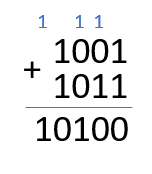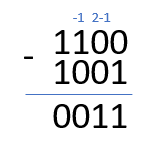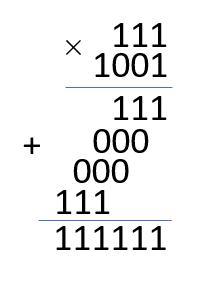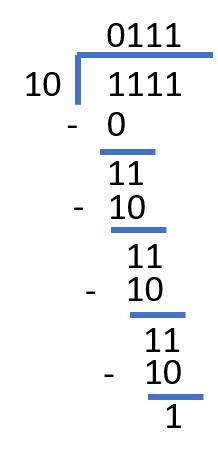Math Calculators
Binary Calculator

# Binary Calculator

Binary calculator for binary to decimal conversion, decimal to binary conversion, binary operations – addition, subtraction, multiplication, division.

101110110

Binary to Decimal 10101010 = 170
Decimal to Binary 170 = 10101010

There was an error with your calculation.This calculator can be used for performing various types of operations with binary numbers. It combines binary addition calculator, binary subtraction calculator, binary division calculator, binary multiplication calculator, and binary conversion calculator. The binary conversion calculator can convert binary values to decimal values and vice versa.

## Directions for use

### Binary Calculations

Use the first part of the calculator to perform binary calculations – addition, subtraction, division or multiplication of two binary numbers. To perform a calculation, enter the given binary numbers and choose the sign of the necessary mathematical operation (+, -, ×, ÷). Then press “Calculate.” The calculator will display the result in binary values, as well as in decimal values.

### Convert Binary Value to Decimal Value

To convert a binary value to a decimal value, use the second part of the calculator. Simply enter the given binary value and press “Calculate.”

### Convert Decimal Value to Binary Value

Use the third part of the calculator to perform decimal to binary conversions. Enter the given decimal value and press “Calculate.” All parts of the calculator work with whole numbers.

## Binary numbers

A binary number consists of only ones and zeros, for example, 10001110101010 would be a binary number. A binary number system is sometimes called a base-2 numeral system, so a binary calculator is a base 2 calculator.

A binary number in the base 2 system is formed in the same way a decimal number is formed in the “normal” base 10 system. In the decimal numeral system, we count 0, 1, 2, 3, 4, 5, 6, 7, 8, 9 … and then we go back to 0, but add a 1 in front of it, getting 10. In the binary system we do the same thing, but we reach 10 much sooner. We count 0, 1 … and now we don’t have any more digits, so we immediately go to 10.

Therefore, 2 in decimal equals 10 in binary. To write 3 in binary, we continue from 10 to 11. But to write 4, we need to go to 00, adding 1 in front. Therefore, 4 in decimal equals 100 in binary. The decimal-binary equivalents of some numbers are presented in the table below.

Decimal Binary
0 0
1 1
2 10
3 11
4 100
5 101
6 110

Note, that just like in the decimal number system, adding zeros in front of the number doesn’t change the value. For example, writing 6 as 06 would technically be correct. Similarly, in binary 6 could be written as 110 or 0110.

## Binary conversions

### Converting decimal numbers to binary numbers

The easiest way to convert a decimal number into a binary number is to continuously divide the given decimal number by 2, and to note the remainders. Once you get 0 as a quotient, write down all remainders in reverse order, to get the binary number. For example, let’s convert 17 to a binary number:

1. 17 ÷ 2 = 8 R1
2. 8 ÷ 2 = 4 R0
3. 4 ÷ 2 = 2 R0
4. 2 ÷ 2 = 1 R0
5. 1 ÷ 2 = 0 R1

Writing down all remainders in reverse order, we will get the following number: 10001. 17₁₀ = 10001₂. (Note, how the order of the numeral system is added as a subscript following the number).

### Converting binary numbers to decimal numbers

To convert a binary value to a decimal value, follow the steps below. For clarity, the steps will include a conversion example. Let’s convert 100101₂ to a decimal number.

1. Start from the left-most digit of the binary number. Multiply the number obtained in the previous step by 2, and add the current digit. In the example of 100101, the left-most digit is 1. We didn’t have any previous step yet, so the previous number is 0: (0 × 2) + 1 = 0 + 1 = 1.
2. Repeat step 1 for the second digit. In the example of 100101, the second digit from the left is 0. The number from the previous step is 1. (1 × 2) + 0 = 2.
3. Repeat step 1 for every consecutive digit. The final sum will be the decimal representation of the given binary number.
1 (0 × 2) + 1 = 1 1
0 (1 × 2) + 0 = 2 2
0 (2 × 2) + 0 = 4 4
1 (4 × 2) + 1 = 9 9
0 (9 × 2) + 0 = 18 18
1 (18 × 2) + 1 = 37 37

Finally, 100101₂ = 37₁₀

## Binary Calculations

Addition rules in the binary system are equivalent to addition rules in the decimal system. The only difference is that the number is carried to the next digit already when the sum reaches 2 (as opposed to 10 in the decimal system). The rules of binary addition are:

• 0 + 0 = 0
• 0 + 1 = 1
• 1 + 0 = 1
• 1 + 1 = 0, and 1 is carried over.

For example,1001 + 1011 = 10100

### Binary subtraction

Binary subtraction also follows the rules of decimal subtraction, with the borrowing from the next-order digit happening when 1 has to be subtracted from 1. The rules of binary subtraction are:

• 0 – 0 = 0
• 1 – 0 = 1
• 1 – 1 = 0
• 0 – 1 = 1, 1 is borrowed.

When you borrow a number from the next-order digit, it essentially becomes 2 for the digit in question, and 2 – 1 = 1. For example,1100 – 1001 = 0011 = 11

In this example, we can’t borrow 1 from the next-order digit, so we have to jump one digit further. Then the second-to-right digit essentially becomes 2, and when we borrow from it, it reduces to 1. The blue numbers on the picture represent digit changes when borrowing.

### Binary multiplication

The rules for binary multiplication are:

• 0 × 0 = 0
• 0 × 1 = 0
• 1 × 0 = 0
• 1 × 1 = 1

For example,### Binary division

Binary division follows the same rules as long division for decimal numbers. Similarly to the decimal system, in the binary numeral system, division by 0 cannot be performed. The rules for binary division are:

• 0 ÷ 0 cannot be performed
• 0 ÷ 1 = 0
• 1 ÷ 0 cannot be performed
• 1 ÷ 1 = 1

For example, 1111 ÷ 10 = 111 R1:## The Short History of Binary Numbers

The history of binary numbers can be traced back to the late 17th century, when German mathematician and philosopher Gottfried Wilhelm Leibniz introduced this concept. Leibniz was fascinated by the idea of a binary system in which only two symbols represent all numbers. He wrote about this concept in his manuscript, "Explanation of the Binary Arithmetic."

Leibniz's ideas were not widely accepted then. But in the early 20th century, the binary system began to gain widespread recognition and use. The development of electronic computers in the 1940s and 1950s was a major factor in the rise of binary numbers. Computers use binary code to store data and do calculations, and binary numbers are now an essential part of how technology works.

One of the earliest uses of binary numbers in practice was the telegraph's development. In 1801, George Boole, an English mathematician and philosopher, proposed using binary logic for creating the system of electrical switches. This system would allow telegraph operators to send messages more efficiently using a series of on-and-off electrical signals.

The invention of the first electronic computer, the Atanasoff-Berry Computer (ABC), in 1937, was a significant step towards the widespread use of binary numbers. The ABC used binary numbers to represent data and perform calculations. It was the first computer that used electronic switches rather than mechanical ones.

The development of electronic computers in the 1940s and 1950s led to the widespread use of binary numbers.

## Real-life Applications

Binary numbers are used not only in computer science and technology, but also find real application in various other areas of human activity.

Computer memory is made up of transistors, either in an "on" or "off" state. In a binary system, "on" is represented by the number 1, and "off" is represented by the number 0. This allows for data to be stored in binary code, where each "on" or "off" state represents a 1 or 0 in a string of binary digits. For example, a string of eight binary digits, such as "01101001," could represent the letter "Y" in the computer's ASCII code.

Each pixel in a digital image can be represented by a binary digit combination that represents the intensity of a specific color (red, green, blue). In the RGB color model, the color white can be represented by the binary value "111" (7 in decimal), which means that all three color channels (red, green, and blue) are at their maximum intensity. Similarly, the color black can be represented by the binary value "000" (0 in decimal), which means that all three color channels are at their minimum intensity.

In the field of digital communications, data can be transmitted through a channel by mapping each character of a message to binary digits and then sending it as a stream of bits. The receiver can then decode the bits back to the original message.

Digital devices such as computers, smartphones, and televisions use binary code to represent data and perform calculations. This allows them to process and store large amounts of information efficiently.

Binary numbers are used in telecommunications. Binary code transmits data over long distances via telephone lines, cable, and satellite. This allows for faster and more efficient communication, making it possible for us to stay connected across the globe.

Binary numbers control automated machinery such as robots and CNC machines in manufacturing. These machines use binary code to interpret instructions, allowing them to perform precise tasks such as drilling, cutting, and welding.

Binary numbers are also used in the field of medicine. Medical equipment such as CT scanners, MRI, and X-ray machines use binary code to process and analyze medical images.

Binary numbers are also used in the field of transportation. Modern cars use binary code to control various functions such as engine management, air conditioning, and navigation.

The concept of binary numbers, first introduced by Leibniz, has become an essential part of our daily lives. Today, the use of binary numbers is essential to the functioning of modern technology and continues to play an essential role in the development of new technologies.